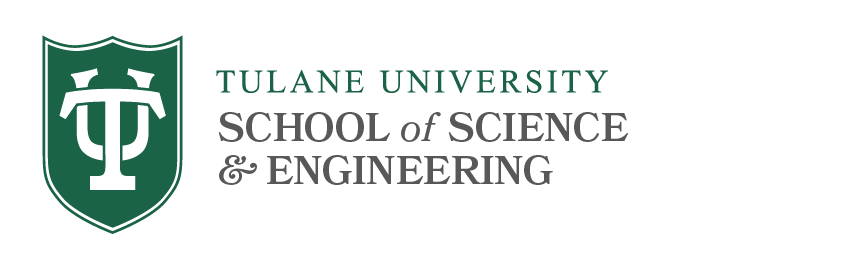# Journals A-D

## A

Abstract and Applied Analysis {electronic only}

Abstracts of the American Mathematical Society {paper only}

ACM Computing Surveys

ACM Transactions on Algorithms {electronic only}

ACM Transactions on Computational Logic {electronic only}

ACM Transactions on Computer-Human Interaction {electronic only}

ACM Transactions on Computer Systems {electronic only}

ACM Transactions on Database Systems {electronic only}

ACM Transactions on Design Automation of Electronic Systems {electronic only}

ACM Transactions on Graphics {electronic only}

ACM Transactions on Information and Systems Security {electronic only}

ACM Transactions on Mathematical Software {electronic only}

ACM Transactions on Modeling and Computer Simulation {electronic only}

ACM Transactions on Programming Languages and Systems {electronic only}

ACM Transactions on Software Engineering and Methodology {electronic only}

ACTA Arithmetica

ACTA Applicandae Mathematicae {electronic only with 1 year delay in access}

ACTA Mathematica

ACTA Mathematica Hungarica

ACTA Mathematica Scientia {electronic only}

ACTA Numerica {electronic only}

ACTA Scientiarum Mathematicarum {paper only}

Advances in Applied Mathematics {electronic only}

Advances in Difference Equations {electronic only}

Aequationes Mathematicae {electronic only}

Algebra Universalis {electronic only}

Algebraic and Geometric Topology {electronic only}

Algebras, Groups and Geometries {paper only}

American Journal of Mathematics

American Mathematical Monthly

American Statistician, The {paper only}

AMSTAT News

Analysis

Annalen der Physik {electronic only}

Annales de l’I.S.U.P. {paper only}

Annales de l’Institut Fourier. Grenoble

Annales de l’Institut Henri Poincare - Analyse non lineaire

Annales de l’Institut Henri Poincare - Section B Probabilites et Statistique

Annales Polonici Mathematici

Annales Scientifiques de l’Ecole normale superieure

Annali della Scuola normale superior di Pisa, Classe di scienze {paper only}

Annali di matematica pura ed applicata {electronic only}

Annals of Applied Probability

Annals of Applied Statistics

Annals of Global Analysis and Geometry {electronic only}

Annals of Mathematics {electronic only}

Annals of Mathematical Statistics [discontinued]

Annals of Probability

Annals of Statistics

Annals of the Institute of Statistical Mathematics

Annals of the New York Academy of Sciences {electronic only}

Anziam Journal, The {electronic only}

Applicable Analysis {electronic only}

Applied Mathematics and Computation {electronic only}

Applied Mathematics and Optimization {electronic only}

Applied Mathematics Letters {electronic only}

Applied Numerical Mathematics {electronic only}

Archiv der Mathematik

Archive for Mathematical Logic {electronic only with 1 year delay in access}

Archive for Rational Mechanics and Analysis {electronic only}

Arkiv fur Matematik

Asian Journal of Mathematics

Asymptotic Analysis

Australian & New Zealand Journal of Statistics {electronic only}

## B

Bernoulli

BIT Numerical Mathematics

Bulletin de la Societe Mathematique de France

Bulletin de la Societe Mathematique de France - Memoire {electronic only}

Bulletin des Sciences Mathematiques {electronic only}

Bulletin for the Belgian Mathematical Society {paper only}

Bulletin (New Series) of the American Mathematical Society

Bulletin of the Australian Mathematical Society {electronic only}

Bulletin of the Brazilian Mathematical Society {electronic only with 1 year delay in access}

Bulletin of the Institute of Mathematical Statistics (IMS)

Bulletin of the Korean Mathematical Society {electronic only}

Bulletin of the London Mathematical Society {electronic only}

Bulletin of the Polish Academy of Sciences, Mathematics

## C

Cahiers de Topologie et Geometrie Differentielle Categoriques

Canadian Journal of Mathematics {paper only}

Canadian Journal of Statistics {electronic only}

Casopis pro Pestoviani matematiky

Chaos {electronic only}

Chaos, Solitons, and Fractals {electronic only}

Classical and Quantum Gravity {electronic only}

Collectanea Mathematica

Colloquium Mathematicum

Combinatorica

Combinatorics, Probability & Computing (CPC) {electronic only}

Commentarii Mathematici Helvetici

Commentationes Mathematicae Universitatis Carolinae {paper only}

Communications in Algebra {electronic only}

Communications in Analysis and Geometry

Communications in Mathematical Physics {electronic only}

Communications in Mathematical Sciences

Communications in Partial Differential Equations {electronic only}

Communications in Statistics: Simulation and Computation {electronic only}

Communications in Statistics: Theory and Methods {electronic only}

Communications on Pure and Applied Mathematics {electronic only}

Complex Variables: Theory and Application

Compositio Mathematica {electronic only}

Comptes Rendus mathematiques de l’Academie des sciences {paper only}

Comptes Rendus. Mathematique

Computational and Applied Mathematics {electronic only}

Computational Geometry {electronic only}

Computational Statistics and Data Analysis {electronic only}

Computers and Mathematics with Applications {electronic only}

Conformal Geometry and Dynamics {electronic only}

Constructive Approximation {electronic only}

Cryptologia {electronic only}

Czechoslovak Mathematical Journal

## D

Differential and Integral Equations {paper only}

Differential Equations

Differential Equations and Nonlinear Mechanics {electronic only}

Differential Geometry and its Applications {electronic only}

Discrete and Continuous Dynamical Systems, Series A {electronic only}

Discrete and Continuous Dynamical Systems, Series B {electronic only}

Discrete Applied Mathematics {electronic only}

Discrete Mathematics {electronic only}

Discrete Optimization {electronic only}